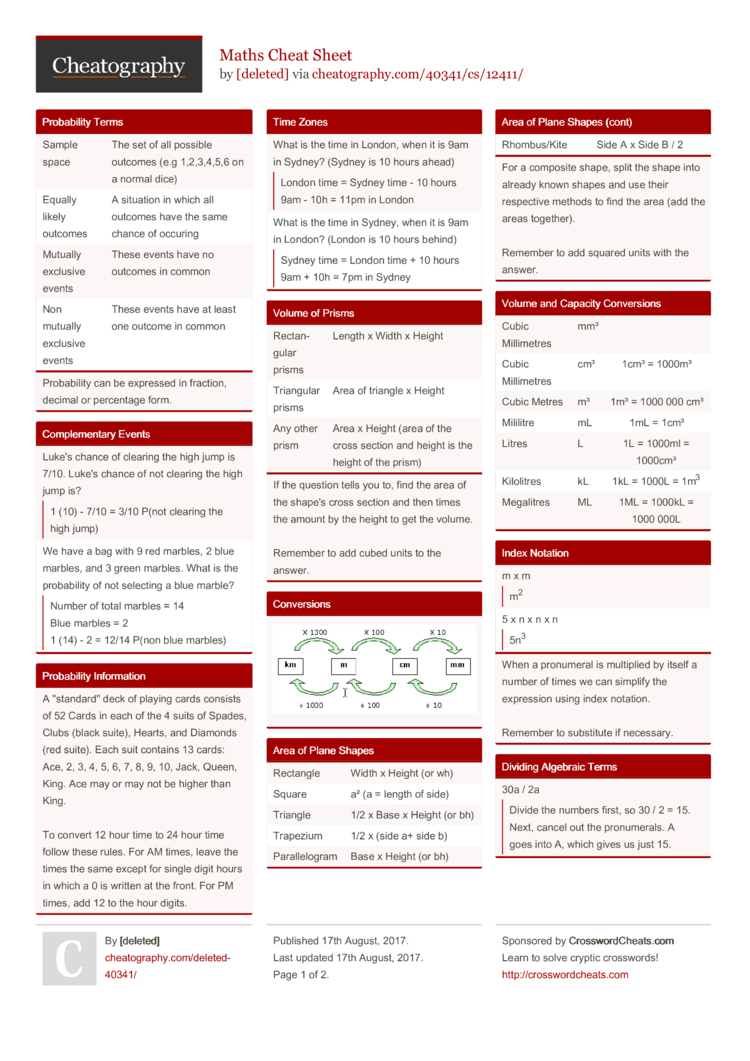# Maths Cheat Sheet by [deleted]

### Probab­ility Terms

 Sample space The set of all possible outcomes (e.g 1,2,3,­4,5,6 on a normal dice) Equally likely outcomes A situation in which all outcomes have the same chance of occuring Mutually exclusive events These events have no outcomes in common Non mutually exclusive events These events have at least one outcome in common
Probab­ility can be expressed in fraction, decimal or percentage form.

### Comple­mentary Events

 Luke's chance of clearing the high jump is 7/10. Luke's chance of not clearing the high jump is?1 (10) - 7/10 = 3/10 P(not clearing the high jump) We have a bag with 9 red marbles, 2 blue marbles, and 3 green marbles. What is the probab­ility of not selecting a blue marble?Number of total marbles = 14 Blue marbles = 2 1 (14) - 2 = 12/14 P(non blue marbles)

### Time Zones

 What is the time in London, when it is 9am in Sydney? (Sydney is 10 hours ahead)London time = Sydney time - 10 hours 9am - 10h = 11pm in London What is the time in Sydney, when it is 9am in London? (London is 10 hours behind)Sydney time = London time + 10 hours 9am + 10h = 7pm in Sydney

### Volume of Prisms

 Rectan­gular prisms Length x Width x Height Triangular prisms Area of triangle x Height Any other prism Area x Height (area of the cross section and height is the height of the prism)
If the question tells you to, find the area of the shape's cross section and then times the amount by the height to get the volume.

### Probab­ility Inform­ation

 A "­sta­nda­rd" deck of playing cards consists of 52 Cards in each of the 4 suits of Spades, Clubs (black suite), Hearts, and Diamonds (red suite). Each suit contains 13 cards: Ace, 2, 3, 4, 5, 6, 7, 8, 9, 10, Jack, Queen, King. Ace may or may not be higher than King.To convert 12 hour time to 24 hour time follow these rules. For AM times, leave the times the same except for single digit hours in which a 0 is written at the front. For PM times, add 12 to the hour digits.

### Index Notation

 m x mm2 5 x n x n x n5n3
When a pronumeral is multiplied by itself a number of times we can simplify the expression using index notation.

Remember to substitute if necessary.

### Dividing Algebraic Terms

 30a / 2aDivide the numbers first, so 30 / 2 = 15. Next, cancel out the pronum­erals. A goes into A, which gives us just 15. 12ab / 6a2Divide the numbers first, so 12 / 6 equals 2. Next, cancel out the pronum­erals. A goes into A but B does not go into A. This gives us 2b / a.
Remember to always write the dividing algebraic terms in fraction form.

### Factor­ising Algebraic Terms

 3a + 123 x a + 3 x 4 is the expanded form. The factorised form is 3(a + 4). 6m + 9First, find the HCF. In this case, it is 3. Put the HCF out the front of a pair of brackets. Find what the HCF is multiplied by to get each term. So we end up getting 3(2m + 3)
Factor­ising is the reverse form of expanding. A good way to check your factor­isation is by expanding your answer it to see if you get the original expression

### Conver­sions### Area of Plane Shapes

 Rectangle Width x Height (or wh) Square a² (a = length of side) Triangle 1/2 x Base x Height (or bh) Trapezium 1/2 x (side a+ side b) Parall­elogram Base x Height (or bh) Rhombu­s/Kite Side A x Side B / 2
For a composite shape, split the shape into already known shapes and use their respective methods to find the area (add the areas together).

### Volume and Capacity Conver­sions

 Cubic Millimetres mm³ Cubic Millimetres cm³ 1cm³ = 1000m³ Cubic Metres m³ 1m³ = 1000 000 cm³ Mililitre mL 1mL = 1cm³ Litres L 1L = 1000ml = 1000cm³ Kilolitres kL 1kL = 1000L = 1m3 Megalitres ML 1ML = 1000kL = 1000 000L

### Adding and Subtra­cting Like Terms

 5x - 2y - 3x + 7yMove the terms with the same pronumeral next to each other. So we get 5x - 3x - 2y + 7y Simplify and you get 2x + 5y 7ab - 3bc + 2abMove the terms with the same pronumeral next to each other. So we get 7ab + 2ab - 3bc Simplify and you get 9ab - 3bc
Only like terms can be added or subtracted together.

### Multip­lying Algebraic Terms

 10 x 3n10 x 3 = 30 30 x n = 30n 20n x 3mn20 x 3 = 60 60 x n x n x m = 60n2m
Remember to multiply the numbers first, then multiply the pro numerals (or add it to the end of the product).

Negative and positive rules also apply to any problems.

### Negative and Positive Rules### Expanding Algebraic Terms

 5(y + 3) + 2y5 x y = 5y 5 x 3 = 15 5y + 2y = 7y Expanded form is 7y + 15 3(a + 4) + 2(5 - a)3 x a = 3a 3 x 4 = 12 2 x 5 = 10 2 x a = 2a 3a +- 2a = a 12 + 10 = 22 Expanded form is a + 22
To write an expression without grouping symbols, multiply each term inside the grouping symbols by the term outside.

Watch out for expres­sions that have negative signs outside the grouping symbols2 Pages
//media.cheatography.com/storage/thumb/deleted-40341_maths.750.jpg

PDF (recommended)